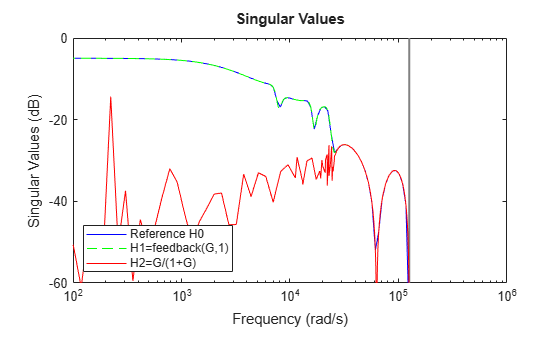Documentation

## Using FEEDBACK to Close Feedback Loops

This example shows why you should always use FEEDBACK to close feedback loops.

### Two Ways of Closing Feedback Loops

Consider the following feedback loopwhere

```K = 2; G = tf([1 2],[1 .5 3])```
```G = s + 2 --------------- s^2 + 0.5 s + 3 Continuous-time transfer function. ```

You can compute the closed-loop transfer function `H` from r to y in at least two ways:

• Using the `feedback` command

• Using the formula

`$H=\frac{G}{1+GK}$`

To compute `H` using `feedback`, type

`H = feedback(G,K)`
```H = s + 2 --------------- s^2 + 2.5 s + 7 Continuous-time transfer function. ```

To compute `H` from the formula, type

`H2 = G/(1+G*K)`
```H2 = s^3 + 2.5 s^2 + 4 s + 6 ----------------------------------- s^4 + 3 s^3 + 11.25 s^2 + 11 s + 21 Continuous-time transfer function. ```

### Why Using FEEDBACK is Better

A major issue with computing `H` from the formula is that it inflates the order of the closed-loop transfer function. In the example above, `H2` has double the order of `H`. This is because the expression `G/(1+G*K)` is evaluated as a ratio of the two transfer functions `G` and `1+G*K`. If

`$G\left(s\right)=\frac{N\left(s\right)}{D\left(s\right)}$`

then `G/(1+G*K)` is evaluated as:

`$\frac{N}{D}{\left(\frac{D+KN}{D}\right)}^{-1}=\frac{ND}{D\left(D+KN\right)}.$`

As a result, the poles of `G` are added to both the numerator and denominator of `H`. You can confirm this by looking at the ZPK representation:

`zpk(H2)`
```ans = (s+2) (s^2 + 0.5s + 3) --------------------------------- (s^2 + 0.5s + 3) (s^2 + 2.5s + 7) Continuous-time zero/pole/gain model. ```

This excess of poles and zeros can negatively impact the accuracy of your results when dealing with high-order transfer functions, as shown in the next example. This example involves a 17th-order transfer function `G`. As you did before, use both approaches to compute the closed-loop transfer function for `K=1`:

```load numdemo G H1 = feedback(G,1); % good H2 = G/(1+G); % bad```

To have a point of reference, also compute an FRD model containing the frequency response of G and apply `feedback` to the frequency response data directly:

```w = logspace(2,5.1,100); H0 = feedback(frd(G,w),1);```

Then compare the magnitudes of the closed-loop responses:

```h = sigmaplot(H0,'b',H1,'g--',H2,'r'); legend('Reference H0','H1=feedback(G,1)','H2=G/(1+G)','location','southwest') setoptions(h,'YlimMode','manual','Ylim',{[-60 0]})```The frequency response of `H2` is inaccurate for frequencies below 2e4 rad/s. This inaccuracy can be traced to the additional (cancelling) dynamics introduced near z=1. Specifically, `H2` has about twice as many poles and zeros near z=1 as `H1`. As a result, `H2(z)` has much poorer accuracy near z=1, which distorts the response at low frequencies. See the example Using the Right Model Representation for more details.